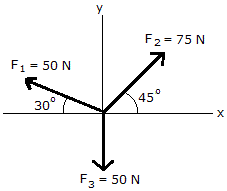# Engineering Mechanics - Force Vectors - Discussion

### Discussion :: Force Vectors - General Questions (Q.No.2)

2.Determine the magnitude of the resultant force by adding the rectangular components of the three forces.

 [A]. R = 29.7 N [B]. R = 54.2N [C]. R = 90.8 N [D]. R = 24.0 N

Explanation:

No answer description available for this question.

 Subbarayudu said: (Nov 19, 2010) How it comes?

 L.Karthik said: (Dec 9, 2010) How is it possible?

 Syue said: (Apr 19, 2011) How come the answer get A. ? I get D FOR the answer. Please give me the solution. I really need the solution.

 Monica Ann said: (Jun 18, 2011) Solution: First, we must add/ sum up all the forces for x-components. That is... Fx(Rx)= F1x + F2x + F3x (since F3 is parallel to y-axis,F3x=0) = -50cos30 + 75cos45 + 50cos90 Fx(Rx)= 9.7317...N Then, add/sum up all the forces for y-components. Fy(Ry)= F1y + F2y + F3y = 50sin30 + 75sin45 - 50sin90 Fy(Ry)= 28.03300...N R=*squareroot of [(Ry)^2 + (Rx)^2] =*suareroot of [(28.03300859 N)^2 + (9.7317384 N)^2] R=29.67416895 N θ= arctan [Ry/Rx] = arctan [28.03300859/9.7317384] θ= 70.86 degrees Therefore, the answer is A.

 Aamir said: (Jun 9, 2012) Its an long method. Is there any shortcut of it.

 John said: (Jun 26, 2012) I get answer A. We divide the components in to horizontal and vertical components considering by respective axis.

 Cj Manglalan said: (Jul 22, 2012) Get the x and y components of F1, F2 and F3 f1x = 50(cos 30)=43.301 ; f2x = 75 (cos 45) = 53.033 f1y = 50(sin 30)=25 ; f2y = 75 (sin 45) = 53.033 f3 = 50 (since f3 is parallel to y axis) Summation along x = 53.033 - 43.301 = -22.426 Summation along y = 25 + 53.033 - 50 = 28.033 R = SQUARE ROOT OF (((22.426)square) + (((28.033)square)) R= 29.7

 Sadiq said: (Jul 28, 2012) Fx=F1x+F2x+F3x=-50cos30+75cos45-50cos90=9.73n Fy=F1y+F2y+F3y=50sin30+75sin45-50sin90=28.03n Resultant force is, R=square root of Fx^2+Fy^2. You will get : 29.67. R=29.67.

 Anand Reddi said: (Aug 7, 2012) F2x=53.03 F2y=53.03 F1x=-43.3025 F1y=25 F in y direction=-50 By adding horizontal and vertical components then we get Resultant force F=9.7275i+28.03j Magnitude is Sqrt((9.27^2)+(2803^2))==29.669936

 Rajesh said: (Aug 23, 2012) To resolve the all forces in cosine and sin components...like R cos@=75cos45+50cos150+50cos270 R cos@=9.732 similarly R sin@=28.037 Resultant is the square root of the sum of the square of the component... R=29.7N

 Prince Tetteh said: (Feb 10, 2015) The algebraic sum of vertical components of forces should be zero as well as horizontal forces. Sum of vertical component = 75sin45+50sin30-50 = 28.033N. Sum of horizontal component = 75cos45-50cos30 = 9.7317N. Then magnitude of resultant = Root of the (Sum of vertical component squared+Sum of horizontal component squared) = 29.6741N.

 V Thimmaraju said: (Feb 16, 2016) I like this type of answers and I want many more problems and answers.

 Seth said: (May 7, 2017) @Anand. I am getting 43.3012709 how did you get that figure? Please explain it.

 Pravin said: (Apr 22, 2021) Why can we not take the angle of F1 from the positive X-axis (150°) if I take 150° for F1 that would be wrong?

 J.Bhargav Sai said: (May 3, 2021) Fx = - 50cos30 + 75cos45 + 50cos90 Fx = 9.73N. Fy = 50sin30 + 75sin45 - 50sin90 Fy = 28.033N. R = √{(Fx)^2 + (Fy)^2}, R = 29.723N.

 Raymond said: (Sep 18, 2021) How did you get cos90?